﻿ A Shared Parameter Model for Longitudinal Data with Missing Values

### A Shared Parameter Model for Longitudinal Data with Missing Values

Ahmed M. Gad, Nesma M. M. DarwishOPEN ACCESSPEER-REVIEWED

## A Shared Parameter Model for Longitudinal Data with Missing Values

Ahmed M. Gad1,, Nesma M. M. Darwish1

1Department of Statistics, Faculty of Economics and Political Science, Cairo University, Cairo, Egypt

### Abstract

Longitudinal studies represent one of the principal research strategies employed in medical and social research. These studies are the most appropriate for studying individual change over time. The prematurely withdrawal of some subjects from the study (dropout) is termed nonrandom when the probability of missingness depends on the missing value. Nonrandom dropout is common phenomenon associated with longitudinal data and it complicates statistical inference. The shared parameter model is used to fit longitudinal data in the presence of nonrandom dropout. The stochastic EM algorithm is developed to obtain the model parameter estimates. Also, parameter estimates of the dropout model have been obtained. Standard errors of estimates have been calculated using the developed Monte Carlo method. The proposed approach performance is evaluated through a simulation study. Also, the proposed approach is applied to a real data set.

### At a glance: Figures

1
Prev Next

• Gad, Ahmed M., and Nesma M. M. Darwish. "A Shared Parameter Model for Longitudinal Data with Missing Values." American Journal of Applied Mathematics and Statistics 1.2 (2013): 30-35.
• Gad, A. M. , & Darwish, N. M. M. (2013). A Shared Parameter Model for Longitudinal Data with Missing Values. American Journal of Applied Mathematics and Statistics, 1(2), 30-35.
• Gad, Ahmed M., and Nesma M. M. Darwish. "A Shared Parameter Model for Longitudinal Data with Missing Values." American Journal of Applied Mathematics and Statistics 1, no. 2 (2013): 30-35.

 Import into BibTeX Import into EndNote Import into RefMan Import into RefWorks

### 1. Introduction

In longitudinal studies each subject is measured repeatedly for the same response variable at different times or different condition or both. For example, if the weights of a sample of individuals are measured once a week for twenty consecutive weeks, the collection of these weights is longitudinal data. The main advantage of longitudinal studies is that it can distinguish changes over time within individuals and enabling direct study of that change.

Longitudinal data are very common in biomedical research and clinical trials where some of measurement of a person develops over time, for example the status of a disease of one person or the value of a car, evolves or develops over time. In these cases one variable is the underlying characteristic or measurement. Longitudinal studies are in contrast to cross–sectional studies in which single outcome are measured for each individual (taken at only one fixed point in time).

Missing data are very common with longitudinal studies. The missing data occur whenever, one or more of, measurement sequences are incomplete. The missing values could be for many reasons. Missing data can be categorized into two different patterns; intermittent missing pattern and dropout pattern. In intermittent pattern a missing value could be followed by an observed value. Dropout means a missing value never followed by an observed value.

A distinguishing feature of incomplete longitudinal data analysis is the need to address the underlying causes of missing values. Missing data mechanism is classified to three different types due to [1, 2]. These types are missing completely at random, missing at random, and nonrandom missingness. A nonresponse process is missing completely at random (MCAR) if the missingness is independent of both unobserved and observed data, and missing at random (MAR) if, conditional on the observed data, the missingness is independent of the unobserved measurements. A process that is neither MCAR nor MAR is nonrandom (MNAR). For likelihood inference, and when the parameters describing the measurement process are functionally independent of the parameters describing the missingness process, MCAR and MAR are ignorable, in which case the missingness process can be ignored when interest is in inference for the longitudinal process only. The missing data mechanism is referred to as informative if the probability of missingness is related to the underlying response process . Follmann and Wu  have shown that informative missing mechanism is a special case of the nonignorable missing mechanism.

Ignoring the missing values with longitudinal data analysis would lead to biased inference. Many authors have tried to model jointly the response process and the missing data process. This modeling framework includes the selection models, pattern mixture models and shared parameter models. In shared parameter models a random effect is shared between the repeated measures model and the missing data mechanism model.

Many authors have proposed a shared parameter model for longitudinal data subject to informative missingness. Wu and Carroll [3, 5] proposed a model for continuous normally distributed longitudinal data. Follmann and Wu [4, 6, 7, 8, 9] proposed models for binary longitudinal responses. Albert and Follmann  proposed methodology for longitudinal count data.

The EM algorithm  can be used to obtain the maximum-likelihood estimates for incomplete data. However, in the nonrandom case, the simplicity of the EM algorithm is lost. The expectation step is problematic and does not admit a closed form solution. Also, in some situations, the M-step is computationally unattractive. Many authors have tried to introduce new variants of the EM algorithm that can overcome the complexity of the E-step. A possible solution for the intractable E-step is to use the Monte Carlo EM algorithm [12, 13] and a stochastic version of the EM algorithm [13-18]. A relatively recent review of the EM algorithm and its extensions is in  and references therein. The stochastic EM (SEM) algorithm is a stochastic version of the EM algorithm, which has been introduced by , and subsequently in , as a way for executing the E-step using simulation.

The EM algorithm does not provide directly the standard errors of the estimates. Hence, methods for evaluating these standard errors need to be considered. Several methods have been introduced to solve this problem, see for example, Louis [20, 21, 22]. Efron  and  have introduced a stochastic version of the Louis’ method (the Monte Carlo method).

In the current paper, we propose a model in which a random effect is shared between the response process and the missing data mechanism. We develop the stochastic EM algorithm (SEM algorithm) to estimate the model parameters. Also, the Monte Carlo method is developed to obtain the standard errors. In Section 2 we discuss the motivating opiate clinical trial example. In Section 3, we develop the random effects transition model and discuss parameter estimation in Section 4. We illustrate this methodology with the opiate clinical trial data in Section 5. A discussion follows in Section 6.

### 2. The Models

Assume that the number of subjects is m and the intended measurements for the ith subject are ni . Assume that due to missing data only no measurements are available of the ni, whereas nim measurements are missing, ni=nio+nim. Let yij represents the jth measurement on the ith subject, i=1, . . . , m, j=1, …, ni. Let Yi be an ni×1 vector containing the responses that would be obtained, for the ith subject, if there were no missing values. Assume that the observed and missing components of Yi are denoted as Yi,obs and Yi,mis, respectively. Let Ri be a vector of missingness indicators. For a particular realization of (Yi,Ri ), each element of Ri takes a value of one if the corresponding value of Yi is observed and the value of zero if the corresponding value of Yi is missing. In notation;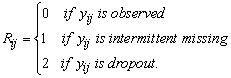It is common to jointly model the response process and the missingness process. The complete data of the ith subject can be viewed as (Yi,obs,Yi,mis,Ri), and the full density function is f(Yi,obs,Yi,mis,Ri| θ, ψ), where the parameters vectors θ and ψ describe the measurement and missingness processes, respectively.

The selection model and pattern mixture model are different factorization of the full density function f(Yi,obs,Yi,mis,Ri| θ, ψ). The selection model framework is based on the factorization;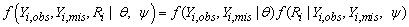The first factor is the marginal density of the measurement process and the second one is the density of the missingness process, conditional on the response. The pattern-mixture model (Little, 1994) is based on the factorization;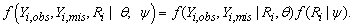This can be seen as a mixture of different populations, characterized by the observed pattern of missingness. Instead of using the selection model or pattern-mixture model, the measurement and the missingness process can be jointly modelled by using a shared-parameter model [3, 5, 6]. These models assume that there is a vector of random effects bi, that is shared between the response and missingness process.

Different missing data mechanisms defined by  can be defined according to the conditional distribution f(Ri | Yi,obs,Yi,mis, ψ). The missing data mechanism is MCAR if f(Ri | Yi,obs,Yi,mis, ψ)= f(Ri | ψ), the missing data mechanism is MAR if f(Ri | Yi,obs,Yi,mis, ψ)= f(Ri | Yi,obs, ψ), otherwise the missing data mechanism is MNAR.

The shared parameter model assume that the response process Yi and the missing data mechanism indicator Ri are conditionally independent of each other, given a group of parameters, bi. Hence the density function of the complete data f(Yi,obs,Yi,mis,Ri| θ, ψ) can be written as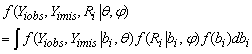Shared parameters bi affect both the response Yi and the missing data indicator Ri, thus can be either observable variables (e.g., gender) or latent variables.

Assuming that the response variable Yi is continuous so, the mixed effects model assumes that the response vectors Yi satisfies the linear regression model;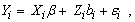where Xi is a set of explanatory variables (design matrix),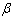is a p× 1 vector of fixed effect parameter, Zi is the random effects covariates and bi is a shared parameter. The shared parameters bi are assumed to be normally distributed with a zero mean and a variance equal to σ2. The errors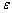i are assumed to be independent normally distributed with zero means and Vi covariance matrix. The matrix Vi may be unstructured and hence it contains ni(ni+1)/2 parameters. Also, the covariance matrix may have a parametric structure, i.e. its elements are functions of a smaller number (vector) of parameters α. In this case it can be written as Vi (α). The main reason for modelling the covariance matrix, Vi, as a function of parameters α is to examine different covariance structures, and for parsimony.

Tsonaka et al  have shown that the shared parameters model, by construction, implies a missing not at random (MNAR) mechanism. The conditional distribution f(Ri | Yi,obs,Yi,mis, ψ) can be viewed as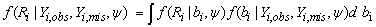which shows that the probability of nonresponse depends on Yi,mis through the posterior f(bi | Yi,obs,Yi,mis, ψ), corresponding therefore to a nonignorable mechanism.

The missing data process, conditional on the random effects bi, can be modeled as ,(1)

where wkij are vectors of covariates and ηk are their corresponding regression coefficients. Also, the parameters γk relate the missingness process (intermittent or dropout) to the response process.

The likelihood function for the parameters (θ, ψ),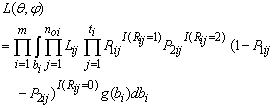(2)

where noi is the number of observed measurements for subject i, ti is the last measurement time, Plij=P(Rij=l|bi,Rij-1≠2), l=1,2 are as given in Eq. (1) and I(.) are indicator function which equal 1 if the condition is met and zero otherwise. Note that,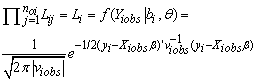where viobs and Xi is a suitable partition of the Vi and Xi respectively and θ=(β,α).

Maximizing this likelihood function we can obtain the parameter estimates. However, this maximization is not easy to implement and computationally intractable. We suggest and develop the stochastic EM algorithm to obtain the parameters estimates.

### 3. Estimation

We propose fitting the shared parameters model using the stochastic EM algorithm. Gad and Ahmed  proposed and developed this algorithm in selection models context. In the shared parameters model context the complete data are Yiobs, Yimis, Ri and bi. In the S-step we need to simulate from the missing data distribution given the observed data, i.e. the conditional distribution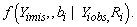This distribution can be partitioned as;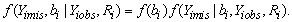Hence, to simulate from this conditional distribution of the missing data given the observed data, we need to simulate from two distributions. First, we simulate from the marginal distribution of bi, f(bi). This is a normal distribution. Second, we simulate from the conditional distribution of the missing data, f(Yimis|bi,Yiobs,Ri). We argue that this simulation can be performed from the conditional distribution f(Yimis|bi,Yiobs,), since the missing data depend on the missing data indicator through the random effect parameter. This distribution now is a normal distribution. The developed EM algorithm iterates two main steps; the stochastic step (S-step) and the maximization step (M-step). Henc at the (t+1)th iteration iterates the following steps.

S-step:

This step consists of two sub-steps; sub-step I and sub-step II.

Sub-step I: For each subject i a single draw is obtained from the marginal distribution of bi; f(bi2(t)). This distribution is the normal distribution with mean zero and variance σ2.

Sub-step II: The missing values of each subject is simulated from the conditional distribution f(Yimis|Yiobs,bi(t),ψ(t)). Note that σ2(t), θ(t) and ψ(t) are the current parameters estimates. In case of the dropout pattern we can simulate the first missing value only for each subject. The remaining missing values in this case can be assumed missing completely at random.

M-Step:

The M-step consists of two sub-steps, the logistic step (M1-step) and the normal step (M2-step). In the M1-step, the maximum likelihood estimates of the dropout parameters in model are obtained using any iterative method for likelihood estimation of binary data models (see, for example ). In the M2-step, the maximum likelihood estimates of the parameters β and α are obtained using an appropriate optimization approach for incomplete data. We recommend using the Jennrich-Schluchter algorithm .

### 4. Standard Errors

Louis  suggest that the information matrix can be approximated by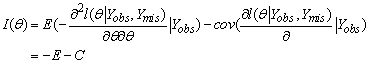(3)

where θ is fixed at the stochastic EM estimates and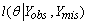is the log-likelihood function.

Evaluating the integrals in the formula in Eq. (3), in the current setting, may not be easy. Efron , also in Ip , suggest using simulation (the Monte Carlo method) to approximate the integrations in Eq. (3). The missing values are simulated from their conditional distribution and then integrations are evaluated by their empirical versions.

The main idea is to simulate M identically distributed samples, q1, q2, …., qM from the conditional distribution of the missing values given the observed values and the parameters estimates, f(Ymis|Yobs,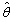). Hence the Louis formula (3) can be approximated by its empirical version, i.e.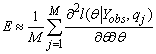and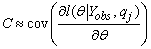The Monte Carlo method is developed to find standard errors of the stochastic EM estimates of parameters. The main idea is to simulate M independent identically distributed samples from the conditional distribution of the missing data given the observed data. Hence, we simulate q1,q2, …,qM samples from the conditional distribution f(Yimis|bi,Yiobs,Ri) and h1,h2, …,hM from the conditional distribution f(bi). Then the two parts in the right hand side of the formula (3) can be approximated by their empirical versions. In notation,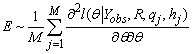and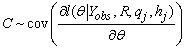where the parameters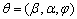is fixed at the SEM estimates,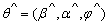.

Having the M pseudo-complete data, the first and second order derivatives of the log-likelihood function are evaluated for each sample, and then it is possible to calculate the quantities E and C and hence the information matrix. The inverse of the information matrix is the covariance matrix of the stochastic EM estimates. The standard error estimates are the square root of the main diagonal elements of this matrix.

### 5. Application (Anti-Depressant Trial)

This data set is taken from a multicenter clinical trial on the treatment of depression. In each of six centers subjects were randomized to one of three treatments, approximately 20 subjects receiving each treatment in each center. The total number of subjects was 367. Each subject was rated on the Hamilton depression score (HAMD); a sum of 16 test items producing a response on a 0-50 scale. Measurements were made on each of five weekly visits. The first measurement made before the treatment and the remaining four measurements made during treatment. Dropout occurs from the third measurement onwards. At the end of the trail 123 (33%) subjects had left.

A subset of these data have been analyzed by , who considered several analyses, including a maximum likelihood analysis. They have shown that an ante-dependence covariance structure of order 2, AD(2), is appropriate for these data.

Diggle and Kenward  use maximum likelihood analysis for these data using the same covariance structure, AD(2), as in , with a less restricted model for the mean response. For the mean profile, they consider a model in which each center is allowed to have a different intercept and quadratic regression relationships for each treatment group.

Figure 1 shows the set of simple mean profiles, based on the observed data for each center. The figure suggests that there is a nonlinear relationship between mean profiles and time. For this reason  suggest modeling the mean profile using quadratic regression for each treatment group.

Figure 1. Observed mean response profiles from antidepressant data trail for each center: (A) center 1; (B) center 2; (c) center 3; (D) center4; (E) center 5; (F) center 6

The set of profiles for the completers at each center are plotted in Figure 2. Several aspects can be concluded from this figure. First, there is typical decrease of HAMD score over time in all the centers. Second, the dispersion of measurements between subjects at week 5 higher than week 1. Third, in center 2, there is a subject stars and remains at a high value.

Figure 2. Observed measurements of antidepressant data and centers: : (A) center 1; (B) center 2; (c) center 3; (D) center4; (E) center 5; (F) center 6

Table 1 shows number of subjects who dropout at each treatment in each center. The positive and negative columns shows number of subjects with positive and negative score increment, respectively, who dropout over the trial period. Center 6 has the highest number of dropouts.

Generally subjects with negative score increments tend to dropout except for few subjects. Dropout occurs consistently from week 3 to week 5, except in the second treatment in center 6. In general week 3 has the highest number of dropouts.

#### Table 2. The stochastic EM estimates and their standard errors for antidepressant data

The proposed model is used for these data. The responses are modeled as: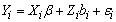where Yi is the antidepressant measures, Xi is a design matrix,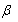is a vector of unknown parameters represent the centers effect in addition to a constant parameter;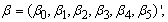bi is the random effects and Zi is a design matrix associated with the shared parameters.

#### Table 3. The stochastic EM estimates vs. true parameters values for the simulated data

The dropout process is modeled according to the model in Eq. (1). Note that the dropout can be considered as a special case of Eq. (3). The model is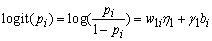The developed stochastic EM algorithm has been applied to the models. The parameters estimates of the response model and the dropout model are presented in Table 2. These parameter estimates have been obtained for 3000 iteration and stop when the difference between the last two is less than .0001 for Jennrich-Schluchter algorithm.

From the results in Table 2 we conclude that the centers effects are statistically significant. Also, the covariance parameters are highly significant. The shared parameter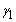is significant which support the nonrandom dropout.

### 6. Simulation Study

The aim of this simulation study is to validate the obtained stochastic EM estimate by comparing them with the true parameters. The simulation setup is as follows. A random effects linear model is used for the response as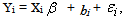whereis a vector consists of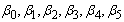. The residualsi are assumed to be independent normally distributed with zero means and covariance matrix Vi. The dropout process is modeled using the logistic model. Hence, we have 6 parameters of the response and the dropout model there is only one parameter (γ).

The developed stochastic EM algorithm is used to find the parameter estimates. The number of iterations is fixed at 3000. The results are presented in Table 3.

Depending on this simulation we can see that the absolute relative bias is small to moderate. The maximum relative bias is around 27%. This means that the proposed approach produce parameters estimates close to the true parameters values. Hence, we can conclude that the proposed approach is reliable and gives reasonable results.

### References

  Rubin, D. B. “Inference and missing data”. Biometrika, 63, 581-592. 1976.In article CrossRef  Little, R.J.A., Rubin, D.B., “Statistical Analysis with Missing Data”,.Wiley, NewYork.1987.In article  Wu, M. C. and Carroll, R. J. “Estimation and comparison of changes in the presence of informative right censoring by modelling the censoring process”, Biometrics, 44, 175-188. 1988.In article CrossRef  Follmann, D. and Wu. M. “An approximate generalized linear model with random effects for informative missing Data”, Biometrics, 51, 151- 168. 1995.In article CrossRef PubMed  Wu, M. C. and Bailey, K. R. “Estimation and comparison of changes in the presence of informative right censoring: conditional linear model”, Biometrics, 45, 939-955. 1989.In article CrossRef PubMed  Ten Have, T. R., Kunselman, A. R., Pulkstenis, E. P., and Landis, J. R. “Mixed effects logistic regression models for longitudinal binary response data with informative drop-out”. Biometrics 54, 367-383. 1998.In article CrossRef PubMed  Pulkstenis, E.P., Ten Have, T. R. and Landis, J. R. “Model for the analysis of binary longitudinal pain data subject to informative dropout through remedication”, Journal of the American Statistical Association, 93, 438-450. 1998.In article CrossRef  Wu, M.C. and Follmann, D. A. “Use of summary measures to adjust for informative missingness in repeated measures data with random effects”, Biometrics, 55, 75-84. 1999.In article CrossRef PubMed  Albert, P. S and Follumann, D.A., “A random effects transition model for longitudinal binary data with informative missigness”, Statistica Neerlandica, 57, 100-111. 2003.In article CrossRef  Albert, P.S. and Follmann, D. A. “Modeling repeated count data subject to informative dropout”, Biometrics, 56, 667-677. 2000.In article CrossRef PubMed  Dempster, A.P., Larid, N.M. and Rubin, D.B., “Maximum likelihood from incomplete data via the EM algorithm (with discussion)”, Journal of Royal Statistical Society B, 39, 1-38. 1997.In article  Tanner, M.A.,Wong,W.H., “The calculation of posterior distributions by data augmentation (with discussion)”, Journal of American Statistical Association, 82, 528-550. 1987.In article CrossRef  Wei, G.C.G., Tanner, M.A., “A Monte Carlo implementation of the EM algorithm and the poor man’s data augmentation algorithm,” Journal Royal Statistical Society B, 55, 425-437. 1990.In article  Celuex, G., Diebolt, J., “The SEM algorithm: a probabilistic teacher algorithm derived from the EM algorithm for the mixture problems,” Computational Statistics Quarterly, 2, 73-82. 1985.In article  Delyon, B., Lavielle, M., Moulines, E., “Convergence of a stochastic approximation version of the EM Algorithm,” Annals of Statistics, 27, 94-128. 1999.In article  Diebolt, J., Ip, E.H.S., “Stochastic EM: method and application”. In: Gilks,W.R., Richardson, S., Spiegelhalter, D.J. (Eds.), Markov Chain Monte Carlo in Practice. Chapman & Hall, London. (Chapter 15). 1996.In article  Gu, M.G., Kong, F.H., “A stochastic approximation algorithm with Markov chain Monte Carlo method for incomplete data estimation problems,” Proc. Natl. Acad. Sci. USA 98, 7270-7274. 1998.In article CrossRef  Zhu, H.T., Lee, S.Y., “Analysis of generalized linear mixed models via a stochastic approximation algorithm with Markov chain Monte Carlo method,” Statist. Comput., 12, 175-183. 2002.In article CrossRef  McLachlan, G.J., Krishnan, T., “The EM Algorithm and Extensions”, Wiley, New York. 1997.In article  Louis, T.A., “Finding the observed information matrix when using the EM algorithm”. Journal of Royal Statistical Society, B 44, 226-232. 1982.In article  Meilijson, I., “A fast improvement to the EM algorithm on its own terms”, Journal of Royal Statistical Society, B 51, 127-138. 1989.In article  Meng, X.L., Rubin, D.B., “Maximum likelihood estimation via the ECM algorithm: a general framework”, Biometrika, 80, 267-278. 1993.In article CrossRef  Efron, B., “Missing data, imputation, and the bootstrap”. Journal of American Statistical Association, 89, 463-475. 1994.In article CrossRef  Ip, E.H.S., “A stochastic EM estimator in the presence of missing data: theory and applications”. Technical Report, Division of Biostatistics, Stanford University, Stanford, California, US. 1994.In article  Tsonaka, R., Verbeke, G. and Lesaffre, E. “A semi-parametric shared parameter model to handle nonmonotone nonignorable missingness”, Biometrics 65, 81-87. 2009.In article CrossRef PubMed  Gad, A.M and Ahmed, A. S. “Analysis of longitudinal data with intermittent missing values using the stochastic EM algorithm”. Computational Statistics & Data Analysis, 50, 2702-2714. 2006.In article CrossRef  McCullagh, P. and Nelder, J. A. “Generalized Linear Models”. 2nd edititon, Chapman and Hall, England. 1989.In article PubMed  Jennrich, R.I., Schluchter,M.D., “Unbalanced repeated measures models with structured covariance matrices”. Biometrika 42, 805-820. 1986.In article  Heyting, A. and Tolboom, J. T. B. M. and Essers, J. G. A. “Statistical handling of dropouts in longitudinal clinical trials”, Statistics in Medicine, 11, 2043-2062. 1992.In article CrossRef PubMed  Diggle, P.J. and Kenward, M.G. “Informative dropout in longitudinal data analysis”, Journal of Royal Statistical Society B, 43, 49-93. 1994.In article